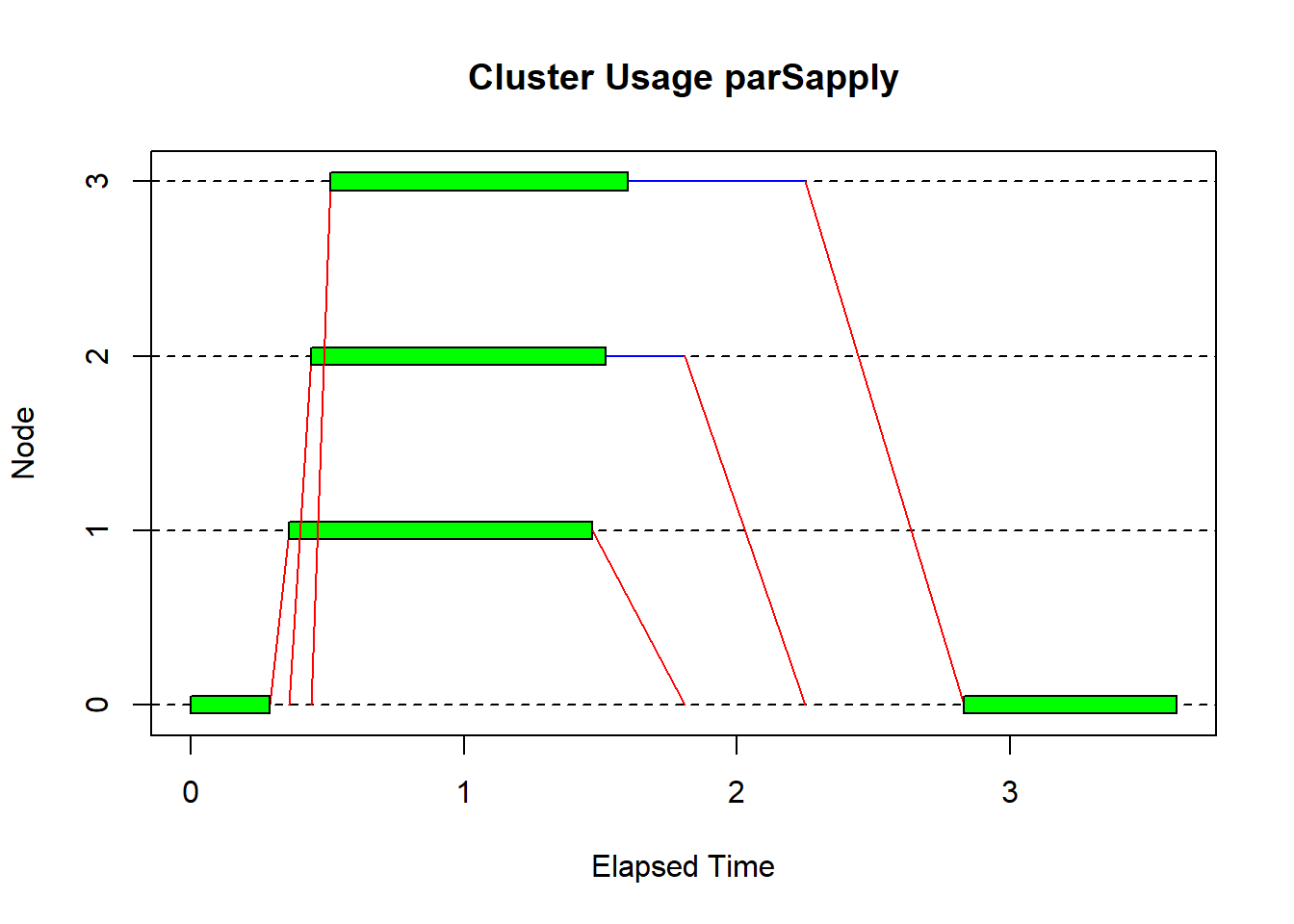What is parallel computing?
How to realize it in R?
When does it make sense?
1. Motivation
2. Theory: Basics
3. Data structure (lapply, sapply, mapply)
4. EXERCISE 1
5. Cluster and cores (parallel, parLapply, parSapply)
6. EXERCISE 2
7. HAVE A BREAK
8. Theory: Runtime and Amdahl’s Law
9. Advanced Parallelism in R (foreach, doParallel)
10. EXERCISE 3
12. Visualize Cluster Usage
13. EXERCISE 4

## Motivation

Disclaimer: We’re talking about explicit parallelism!

Why do we need to parallelise code?

This is what code usually looks like:

N <- 1000
for (i in 1:N){
result <- myFunction(...)
}
doStuff(result,...)

Which can be translated into an example everyone knows from school:

1 worker takes 6 months to build a house.
How long will it take to build 12 houses?

Let’s go parallel!

1 worker takes 6 months to build a house.
How long will 12 workers need to build 1 house?

Therefore: We can obviously improve performance of the overall task by increasing the number of workers/ processors/ threads.

Pro’s and Con’s:

• Less time spent waiting for execution of code
• Less time for coffee during execution
install.packages(c("parallel","snow","doParallel"), dependencies = TRUE,repos = "http://cran.us.r-project.org")

## Theory: Basics

Whenever you do things in parallel, there are some obstacles you need to overcome.

Steps from your sequential source code towards going parallel:

1. Analyse at which point the execution is ‘wasteing’ time.

2. Can this part of code be optimized (in sequential manner)?

3. Can this part of code be parallelized?

Steps 1 & 2 can easily solved by simple analysis of code.

Step 3 is where things become more complex.

You need to ask questions like:

• Is it even possible to go parallel?
• Does it even make sense to go parallel?
• Is there a shared data source?
• Will shared data be altered?
• Is it possible, that 2 threads are dependent on each other?
• Do I need Mutual exclusion? (and which concept should I use?)
• How do I avoid Deadlocks?

• What parallel algorithm concept suits best?

Let’s find out what these questions mean:

Shared data source: I.e. a dataframe used as parameter for functions

Example:

A3: Write back to V B3: Write back to V

If B1 is executed somewhere between A1 and A3 or vice versa. The code will return incorrect data. V = 2 instead of V = 3!

Now imagine you’re putting a lock on each variable while you’re using it.

A3: Wait for W to be unlocked! B3: Wait for V to be unlocked!

## Data Structure

lapply and sapply call a function for each item of a vector or a list

A <- read.table("http://www.trutschnig.net/Datensatz.txt", head=TRUE)
head(A)

Create dataframe to compare the runtime

funct <- c("lapply", "sapply", "parLapply", "parSapply", "doParallel")
runtime <- c(0,0,0,0,0)
compare <- data.frame(funct, runtime)

### Lapply

lapply returns a list

lapply(1:3, function(x) log10(x))

init <- Sys.time()
lapply(A$sum_out, function(x) log10(x)) compare$runtime <- Sys.time() - init

### Sapply

sapply returns a vector or matrix

a = 2

sapply(1:3, function(x) c("log" = log10(x), "quad" = x^a))

init <- Sys.time()
sapply(A$sum_out, function(x) c("quad" = x^a, "log" = log10(x))) compare$runtime <- Sys.time() - init

compare

## Exercise 1

• Import the RTR data from the website
• Create a dataframe where you save the runtimes of sapply, lapply, parSapply, parLapply and doParallel
• Use the functions sapply and lapply to standardise the values of the download speed, sapply should also contain the initial values
address <- url("http://www.trutschnig.net/RTR2015.RData")
head(RTR2015)

Solution

# # Create dataframe
# funct <- c("lapply", "sapply", "parLapply", "parSapply", "doParallel")
# runtime <- c(0,0,0,0,0)
# compare2 <- data.frame(funct, runtime)
#
# # Calculate mean and variance
# avg_dl <- mean(RTR2015$rtr_speed_dl) # sd_dl <- sd(RTR2015$rtr_speed_dl)
#
# # Lapply
# init <- Sys.time()
# lapply(RTR2015$rtr_speed_dl, function(x) (x - avg_dl) / sd_dl) # compare2$runtime <- Sys.time() - init
#
# # Sapply
# init <- Sys.time()
# sapply(RTR2015$rtr_speed_dl, function(x) c("init" = x, "std" = (x - avg_dl) / sd_dl)) # compare2$runtime <- Sys.time() - init
#
# # Print out the comparison matrix
# compare2 

## Cluster and cores

Types of clusters supported by the parallel package:

• PSOCK (Parallel Socket Cluster): creates new empty R sessions (nothing is inherited), default, for all operating systems

• FORK: copies current R session locally (everything is inherited from master process), only UNIX/Mac

### Start and stop cluster

Start and stop cluster with one worker per core

library(parallel)
# get the number of cores available
no_cores <- detectCores(logical = TRUE) - 1
no_cores
##  3
# Initialize cluster
cl <- makeCluster(no_cores, type = "PSOCK")

# Check properties
length(cl)
##  3
cl[]
## node of a socket cluster on host 'localhost' with pid 7412
# do stuff
# (clusterApply, parLapply, parSapply, doParallel ...)

# release bound resources
stopCluster(cl)

### clusterApply

basis for the following functions, input has to be a vector, returns a list

cl <- makeCluster(no_cores)

clusterApply(cl, 1:3, fun = function(x) x^2)
## []
##  1
##
## []
##  4
##
## []
##  9
stopCluster(cl)

### parLapply

input can be a list or a vector, returns a list

cl <- makeCluster(no_cores)

init <- Sys.time()
parLapply(cl, A$sum_out, function(x) log10(x)) compare$runtime <- Sys.time() - init

stopCluster(cl)

### parSapply

input can be a list or a vector, returns a vector or a matrix

cl <- makeCluster(no_cores)

# Get outer value
clusterExport(cl, "a")
# clusterEvalQ(package)

init <- Sys.time()
parSapply(cl, A$sum_out, function(x) c("quad" = x^a, "log" = log10(x))) compare$runtime <- Sys.time() - init

stopCluster(cl)

compare

## Exercise 2

• Calculate the number of cores your computer has and create a cluster
• Use the functions parSapply and parLapply to standardise the values of the download speed
• Compare the runtime of sapply, lapply with parSapply and parLapply, parSapply should also contain the initial values

Solution

# Integrate the parallel library
library(parallel)

# Calculate the number of cores
no_cores <- detectCores() - 1
no_cores

# Create a cluster
cl <- makeCluster(no_cores)

# Export objects from the master to the workers
clusterExport(cl, avg_dl, sd_dl)

# parLapply
init <- Sys.time()
parLapply(cl, RTR2015$rtr_speed_dl, function(x) (x - avg_dl) / sd_dl) compare2$runtime <- Sys.time() - init

# parSapply
init <- Sys.time()
parSapply(cl, RTR2015$rtr_speed_dl, function(x) c("init" = x, "std" = (x - avg_dl) / sd_dl)) compare2$runtime <- Sys.time() - init

# Stop the cluster
stopCluster(cl)

# Compare the runtimes
compare2

# Have a break

### Theory (2)

#### Amdahl’s law

S(n,p) = $$\frac{1}{1-p+(p/n)}$$

S(n,p) := Speedup depending on n and p
n := Number of cores
p := Percentage of parallel code

What are the results from this equation?

• Increasing the percentage of parallel code has more influence on speedup than increasing the number of cores.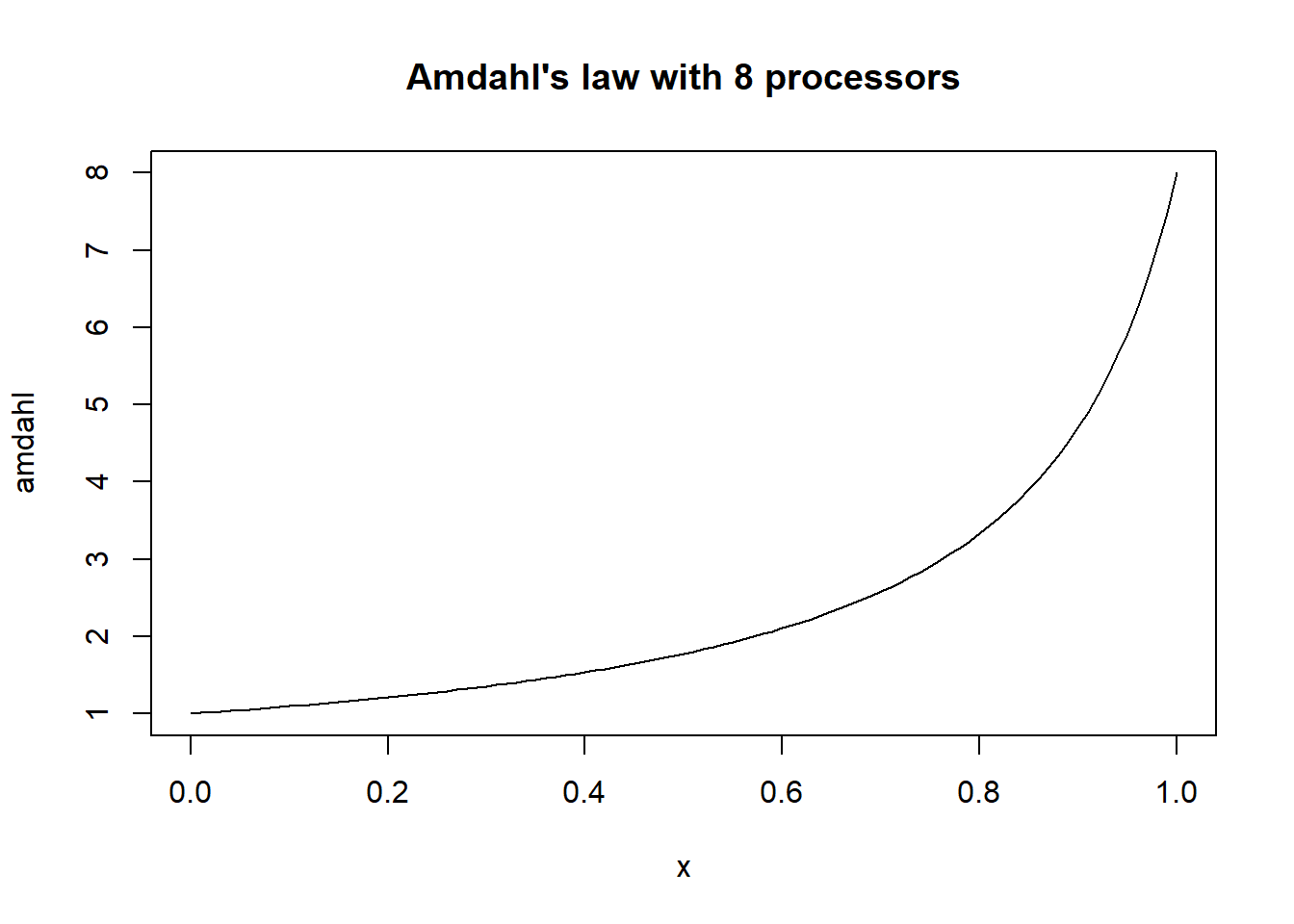• another way for parallel loops
• convenient since different backends are possible
• more beautiful and faster

### foreach

library(foreach)
## Warning: package 'foreach' was built under R version 3.4.4
# Short example for a matrix
foreach(i = 1:3, .export = "a", .combine = rbind) %do% {
c("log" = log10(i), "quad" = i^a)
}
##                log quad
## result.1 0.0000000    1
## result.2 0.3010300    4
## result.3 0.4771213    9

### doParallel

library(doParallel)

cl <- makeCluster(no_cores)

registerDoParallel(cl)

# Check properties
getDoParRegistered()
getDoParWorkers()

# Like sapply using foreach
init <- Sys.time()
foreach(i = A$sum_out, .export = "a", .combine = rbind) %dopar% c("log" = log10(i), "quad" = i^a) compare$runtime <- Sys.time() - init

# Stop cluster
stopCluster(cl)

# Compare runtimes
plot(compare, sub = "Runtime of each function")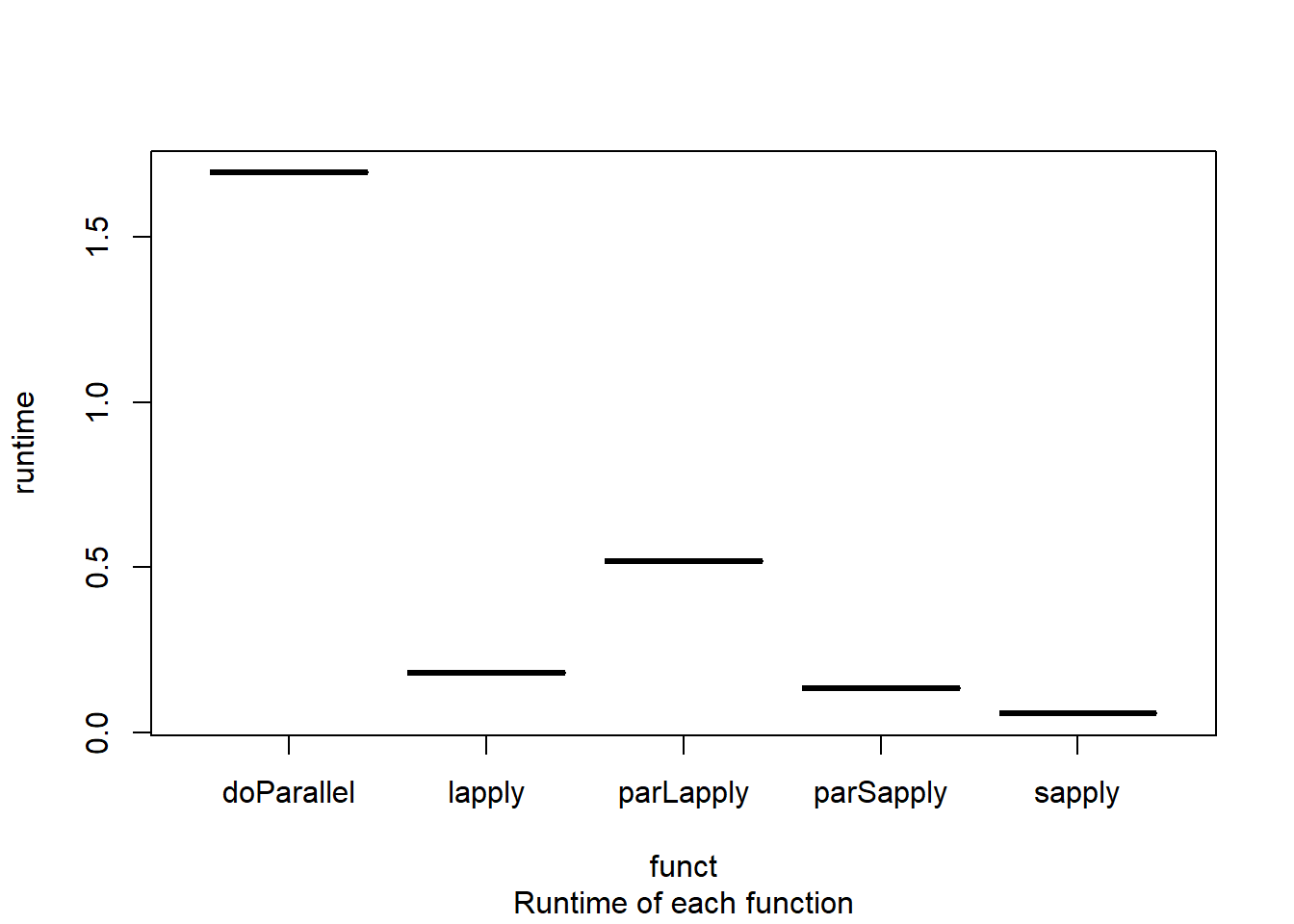## Exercise 3

• Create and register cluster with the library doParallel
• Use the functions foreach and doParallel to standardise the values of the download speed, it should also contain the initial values
• Compare the runtime of foreach with sapply, parSapply with help of a plot

Solution

library(doParallel)

# Create and register cluster
cl <- makeCluster(no_cores)
registerDoParallel(cl)

# Check the properties
getDoParRegistered()
getDoParWorkers()

# foreach doParallel
init <- Sys.time()
foreach(i = RTR2015$rtr_speed_dl, .export = c("avg_dl", "sd_dl"), .combine = rbind) %dopar% { c("init" = i, "std" = (i - avg_dl) / sd_dl) } compare2$runtime <- Sys.time() - init

# Stop cluster
stopCluster(cl)

# Plot runtimes
plot(compare2, sub = "Runtime of each function")

cl <- parallel::makeCluster(no_cores)

parallel::parLapplyLB(cl, A$sum_out, function(x) log10(x)) stopCluster(cl) ### Visualize Cluster Usage Everyone likes graphs, so: How do we visualize cluster usage? Well, we’re using R so there is a package with a suitable function! library("snow") ## Warning: package 'snow' was built under R version 3.4.4 ## ## Attaching package: 'snow' ## The following objects are masked from 'package:parallel': ## ## clusterApply, clusterApplyLB, clusterCall, clusterEvalQ, ## clusterExport, clusterMap, clusterSplit, makeCluster, ## parApply, parCapply, parLapply, parRapply, parSapply, ## splitIndices, stopCluster BUT: As you can see from the messages from loading the package, there are some tradeoffs. Your some functions of parallel-package will be replaced by snow-implementations. Further, the function we use, the snow.time()-function is only an experimental function, without any guarantees! How to use it? isPrime <- function(n){ res <- TRUE for(i in 2:(n-1)){ if((n%%i) == 0) { res <- FALSE break } } res } cl <- parallel::makeCluster(no_cores) seq <- c(10:71) ctime <- snow::snow.time(snow::clusterApply(cl, seq, fun = isPrime)) plot(ctime)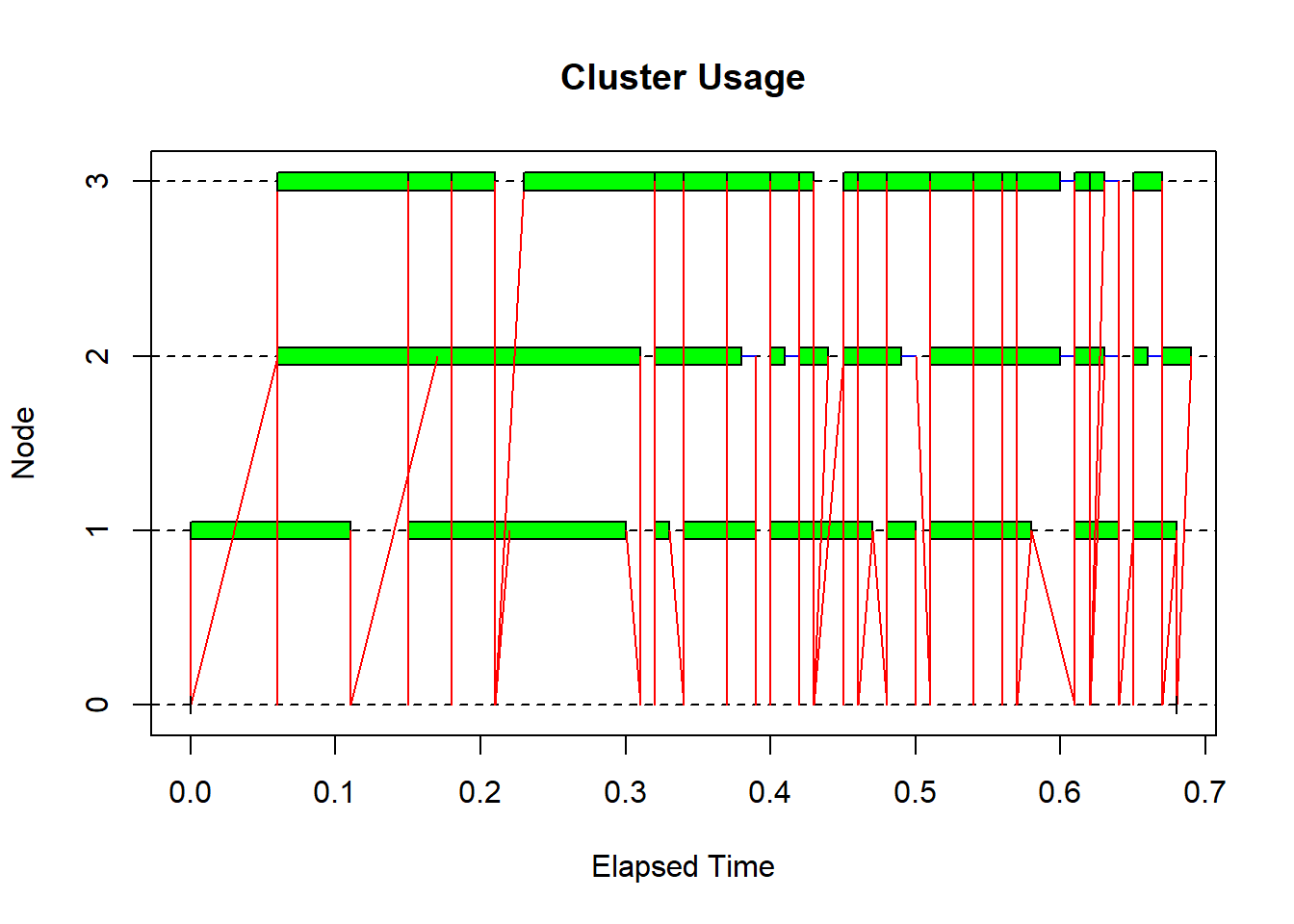ctime ## elapsed send receive node 1 node 2 node 3 ## 0.68 0.11 -0.07 0.58 0.55 0.55 ## Exercise 4 • Use parLapply and parSapply with your solution from ex 2 • Visualize and compare CPU-usage Solution library("snow","parallel") # Calculate mean and variance avg_dl <- mean(RTR2015$rtr_speed_dl)
sd_dl <- var(RTR2015$rtr_speed_dl) # Create a cluster cl <- parallel::makeCluster(no_cores) # Export objects from the master to the workers clusterExport(cl, "avg_dl") clusterExport(cl, "sd_dl") # parLapply ctime <- snow::snow.time(snow::parLapply(cl, RTR2015$rtr_speed_dl, function(x) (x - avg_dl) / sd_dl))
## Warning in UseMethod("as.list"): ungenutzte Verbindung 8 (<-LAPTOP-
## TFH4TRBI:11820) geschlossen
## Warning in UseMethod("as.list"): ungenutzte Verbindung 7 (<-LAPTOP-
## TFH4TRBI:11820) geschlossen
## Warning in UseMethod("as.list"): ungenutzte Verbindung 6 (<-LAPTOP-
## TFH4TRBI:11820) geschlossen
# parSapply
ctimeSap <- snow::snow.time(snow::parSapply(cl, RTR2015\$rtr_speed_dl, function(x) c("init" = x, "std" = (x - avg_dl) / sd_dl)))

# Stop the cluster
stopCluster(cl)

plot(ctime, title = "Cluster Usage parLapply")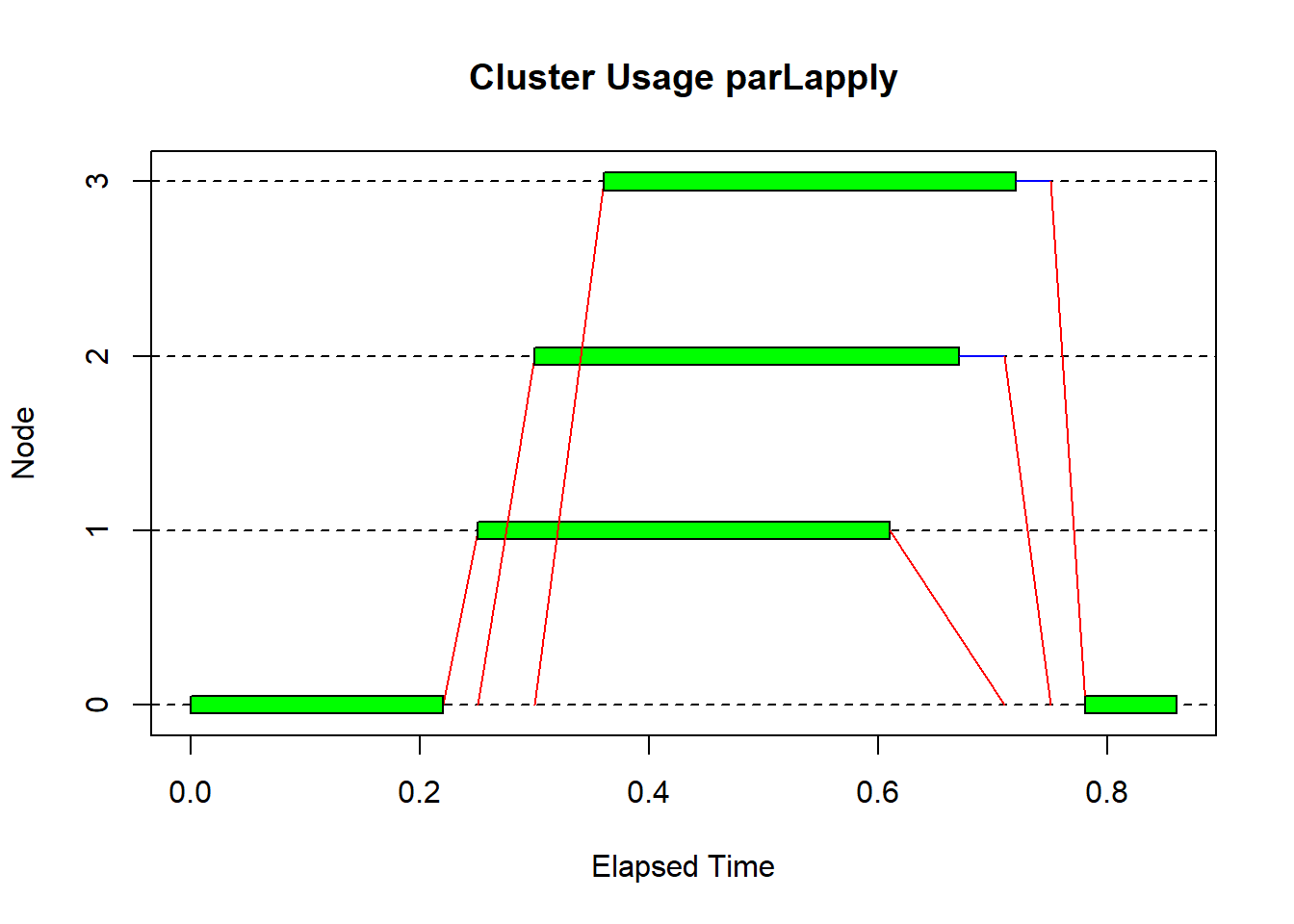plot(ctimeSap, title = "Cluster Usage parSapply")